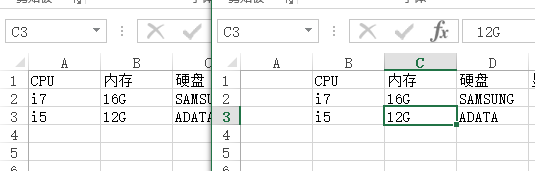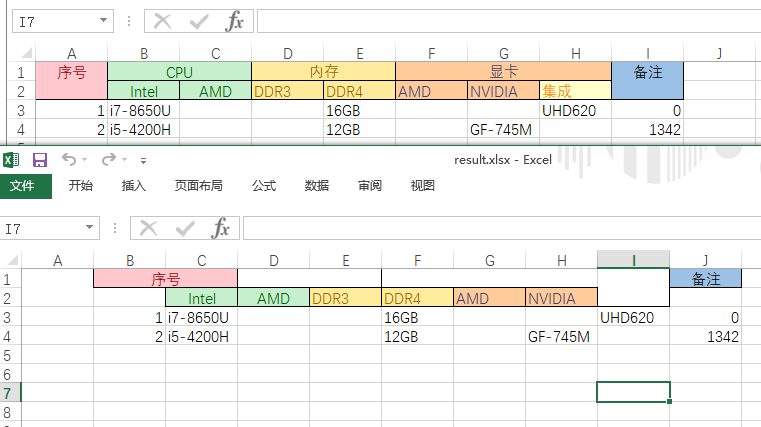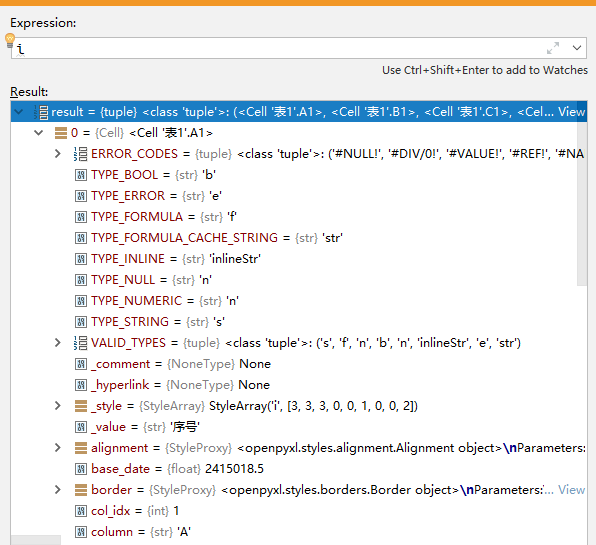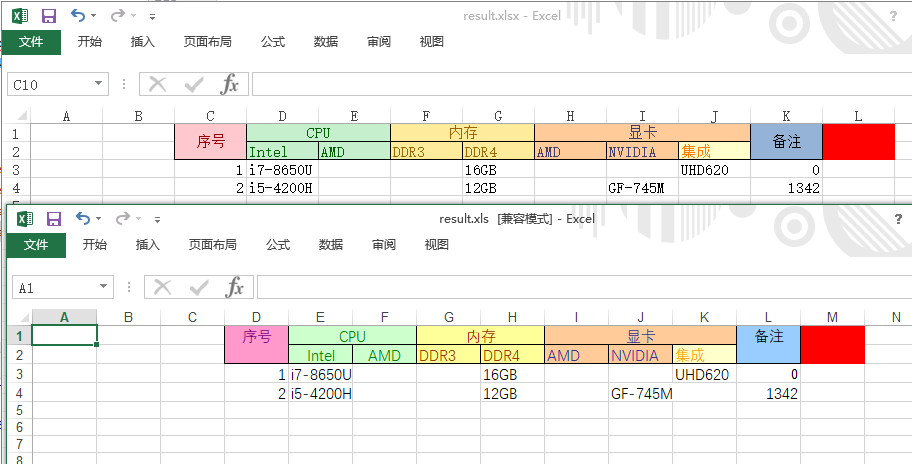• 人们都希望被别人需要 却往往事与愿违
• 你们的时间有限, 所以不要浪费时间活在别人的生活里。Your time is limited, so don't waste it living someone else's [email protected]史蒂夫·乔布斯323254533554544632

# 使用Python给带合并单元格的Excel加一列

7741次浏览 6236字

```import openpyxl

sh = wb.active
sh.insert_cols(1)
wb.save('result.xlsx')
```## openpyxl

### 二十六进制？？

```def twenty_six(coord, size):
# A1:B2, AB1:AC2
left = coord.split(':')
right = coord.split(':')[-1]
left_char = left
right_char = right

if len(left_char) == 1 and len(right_char) == 1:
return "%s:%s" % (chr(ord(left_char + size)), chr(ord(right_char + size)))
elif len(left_char) == 2 and len(right_char) == 2:
return "%s:%s" % (chr(ord(left_char + size)), chr(ord(right_char + size)))
```

```import itertools
import string

table = list(itertools.chain(string.ascii_uppercase,(''.join(pair) for pair in itertools.product(string.ascii_uppercase, repeat=2))))

def twenty_six(char, size):
index = table.index(char)
return table[index + size]
```

```table = [dict(zip(itertools.chain(string.ascii_uppercase,
(''.join(pair) for pair in itertools.product(string.ascii_uppercase, repeat=2))),
[i for i in range(26 * 26)])),
dict(zip([i for i in range(26 * 26)], itertools.chain(string.ascii_uppercase,
(''.join(pair) for pair in itertools.product(string.ascii_uppercase, repeat=2)))))]
```

```base = dict(zip(itertools.chain(string.ascii_uppercase,
(''.join(pair) for pair in itertools.product(string.ascii_uppercase, repeat=2))),
[i for i in range(26 * 26)]))

table = [base, {v: k for k, v in base.items()}]
```

`table`表示从字母查找数字，给数字+2之后在`table`从数字查找字母，调用就更简单了。我爱(ﾉ*･ω･)ﾉ。

### 遍历单元格内容和样式```for i in ws.rows:
for v in i:
print(v.coordinate, v.fill, v.border, v.value)
```

`v.coordinate`表示的是单元格的字母式坐标，我们可以给上面封装好的twenty_six调用下，就能够做好新的移动之后的坐标了。

### 拷贝单元格样式

`ws['A1'].fill='Your fill style'`

```for i in ws.rows:
for v in i:
coord = '移动之后的坐标'
# copy fill border and everything
write_ws[coord].fill = v.fill
write_ws[coord].font = v.border
write_ws[coord].border = v.border
```

`from copy import copy``v.fill`替换成`copy(v.fill)`就好了

### 拷贝单元格内容

```if v.value:
self.write_ws[coord] = v.value
```

```elif type(v.value) == int:
pass
```

```elif isinstance(v.value, float):
pass
```

### 组装……

biu biu biu于是别人给你发了一个xls格式的Excel。

openpyxl不支持xls哦。

(づ￣ 3￣)づ

## xlrd xlwt xlutils

### 读取合并单元格

```for (rlow, rhigh, clow, chigh) in read_ws.merged_cells:
print(rlow, rhigh, clow, chigh)
```

### 写入合并单元格

```for (rlow, rhigh, clow, chigh) in read_ws.merged_cells:
# 2nd & 4th parameter have to -1
write_ws.write_merge(rlow, rhigh - 1, clow + size, chigh - 1 + size, read_ws.cell(rlow, clow).value)
```

### 读取单元格样式

xlrd的样式是怎么读出来的呢？找遍了`sh.cell()`的返回值也没发现有什么结构是存着样式的。

### 应用单元格样式

xlrd的样式不能应用给xlwt哦亲亲。(╯‵□′)╯︵┻━┻气得我当场就把桌子掀了。

```w = XLWTWriter()
w.style_list
```

### 遍历并写入单元格内容和样式

xlrd的`read_ws.nrows``read_ws.ncols`表示了这个表格总计的行数和列数，简单粗暴双循环，同样需要注意布尔值为假的0：

```for i in range(__nrows):
for j in range(__ncols):
# copy style and value
write_ws.write(i, j + size, read_ws.cell(i, j).value, style=__generate_style(i, j))
elif isinstance(read_ws.cell(i, j).value, float):
write_ws.write(i, j + size, read_ws.cell(i, j).value)
```

?是一种神奇的东西，我们每个人都见过，但是却又不知道它的本质，不知道它究竟为何。

?有的时候，可能某些单元格，它是有填充色的，但是内容为空哦。那你这……不就错了吗？

## 这个新的列的表头也得你加

### 自定义前景色

```pattern = xlwt.Pattern()
c.write_wb.set_colour_RGB(0x21, 0, 204, 255)
pattern.pattern = xlwt.Pattern.SOLID_PATTERN
pattern.pattern_fore_colour = 0x21
```

## 结果If you have any further questions, feel free to contact me in English or Chinese.
(6)个小伙伴在吐槽
1. 半夜读着读着手机自动播放音乐，吓我一跳。
尔今夏2018-12-26 01:07 回复
• 小黛：怪我噢?
Benny小土豆2018-12-26 09:17 回复
2. execl功能真是强大啊
汽修软件2018-10-22 14:04 回复
3. 有 Excel 版就会有 OpenDocument 版（笑
布偶君2018-09-25 17:20 回复
• ?这是不可能滴
Benny小土豆2018-09-26 13:54 回复
• 为什么？业界（仮）不使用 OpenDocument？
布偶君2018-09-26 14:03 回复